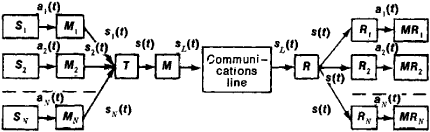# Line Multiplexing

## Line Multiplexing

a method of constructing a communications system in such a way that it provides simultaneous and independent transmission of messages from many senders to an equal number of receivers. In such systems of multichannel communication (multichannel transmission) a common communications line is “multiplexed” into tens or hundreds of individual channels, in each of which an exchange of information occurs between a single pair of subscribers (see Figure 1).Figure 1. Diagram of a system for multichannel transmission of messages: S1S2,..., SN are information sources; a1(t), a2(t),..., aN(t) are messages transmitted by the corresponding information sources; M1, M2,..., MN are individual transmitters (modulators); s1(t), s2(t),..., sN(t) are channel signals produced through conversion by the corresponding information modulators a1(t), a2(t),..., aN(t); T is the channel signal totalizer; s(f) is the group signal, formed by totalizing the channel signals; M is the group transmitter, which converts the group signals s(t) into the linear signal sL(t); R is the group receiver, which converts the linear signal sL(t) into the group signal s(t); R1R2,..., RN are channel, or individual, receivers, which separate from the group signal s(t) the corresponding channel signals s1(t), s2(t),..., sN(t), which are then converted into messages a1(t), a2(t),..., aN(t); MR1, MR2,..., MRN are message receivers

The channel transmitters and the totalizer make up the multiplexing equipment. The group transmitter, the communications line, and the group receiver make up the group transmission path; and the group transmission path, the multiplexing equipment, and the individual receivers make up a multichannel communications system. A necessary and sufficient condition for separability of signals from individual channels is the linear independence of the signals. This condition is expressed mathematically by the identity

C1s1(t) + C2s2 (t) + ⋯ + Cksk(t) + ⋯ + CNsN(t) = 0

This condition is satisfied only in the unique case where all coefficients C are equal to zero simultaneously. Physically, this means that the signal of any channel cannot be formed by a linear combination of signals of all other channels. In practice, multiplexing is achieved by frequency-division, phase-discrimination, amplitude-discrimination, time-division, combination, and structural methods.

The methods most frequently used in multichannel communications systems are frequency division and time division. In frequency-division multiplexing each channel is assigned a certain frequency band within the common pass band of the communications line. On the receiving end, individual frequency filters separate the frequency spectrums of channel signals from the common frequency spectrum of the group signal. In time-division multiplexing (which is a logical development of pulsed communication systems), electronic switches are used to make the communications line or group channel available for transmission of signals in each channel in succession. Similar electronic switches are installed on the receiving end; they, in turn, switch the group channel in the same sequence (synchronously and cophasally), thus connecting it to the corresponding receivers. All channel signals have the same frequency bandwidth but are transmitted sequentially over the communications line. Communications systems using frequency-division or time-division multiplexing are used in main cable lines and radio relay lines.

Multichannel asynchronous-address systems with statistical signal shape recognition multiplexing are promising, particularly for communications among a large number of moving objects (such as aircraft or motor vehicles) and where communication satellites are used in the transmission path. In this system each channel is assigned either a fixed signal shape or a shape varied according to a particular preset program. The signal shape is the recognition sign (or “address”) of each subscriber. The distribution of signals among the channel is achieved by electric shape-matched filters.

### REFERENCES

Nazarov, M. V., B. I. Kuvshinov, and O. V. Popov. Teoriia peredachi signalov. Moscow, 1970.
Dal’niaia sviaz’. Edited by A. M. Zingerenko. Moscow, 1970.

M. V. NAZAROV

Site: Follow: Share:
Open / Close